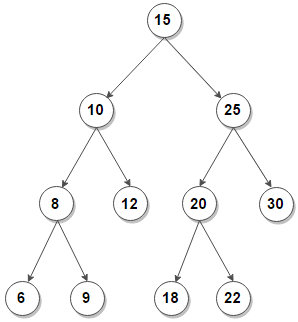# How To Count subtrees in a BST whose nodes lies within a given range?

Given a BST, count subtrees in it whose nodes lies within a given range.

For example, consider below BST. The number of subtrees with nodes in the range [5, 20] are 6.The subtrees are:

6         9          8            10         12         18
/  \          /  \
6    9        8   12
/  \
6    9

Simple solution is to traverse the tree and for each encountered node, check if all nodes under subtree rooted under the node are within the given range or not. The time complexity of above solution is O(n2). We can improve time complexity to linear by traversing the tree in bottom-up manner and transfer some information from children to the parent node.

The idea is to perform post-order traversal on the given BST. Then for any node, if both its left and right subtree are within the range along with the node itself, we can say that the subtree rooted with this node is also within the range. This algorithm can be implemented as follows in C++ where we’re maintaining a reference variable to store the subtrees count –

Output:

The number of subtrees are: 6

The time complexity of above solution is O(n) and auxiliary space used by the program is O(h) for recursion call stack where h is the height of the BST.